# 1.21 Creating a Frequency Table and Histogram in Excel

$$\newcommand{\vecs}{\overset { \rightharpoonup} {\mathbf{#1}} }$$ $$\newcommand{\vecd}{\overset{-\!-\!\rightharpoonup}{\vphantom{a}\smash {#1}}}$$$$\newcommand{\id}{\mathrm{id}}$$ $$\newcommand{\Span}{\mathrm{span}}$$ $$\newcommand{\kernel}{\mathrm{null}\,}$$ $$\newcommand{\range}{\mathrm{range}\,}$$ $$\newcommand{\RealPart}{\mathrm{Re}}$$ $$\newcommand{\ImaginaryPart}{\mathrm{Im}}$$ $$\newcommand{\Argument}{\mathrm{Arg}}$$ $$\newcommand{\norm}{\| #1 \|}$$ $$\newcommand{\inner}{\langle #1, #2 \rangle}$$ $$\newcommand{\Span}{\mathrm{span}}$$ $$\newcommand{\id}{\mathrm{id}}$$ $$\newcommand{\Span}{\mathrm{span}}$$ $$\newcommand{\kernel}{\mathrm{null}\,}$$ $$\newcommand{\range}{\mathrm{range}\,}$$ $$\newcommand{\RealPart}{\mathrm{Re}}$$ $$\newcommand{\ImaginaryPart}{\mathrm{Im}}$$ $$\newcommand{\Argument}{\mathrm{Arg}}$$ $$\newcommand{\norm}{\| #1 \|}$$ $$\newcommand{\inner}{\langle #1, #2 \rangle}$$ $$\newcommand{\Span}{\mathrm{span}}$$$$\newcommand{\AA}{\unicode[.8,0]{x212B}}$$

Once the Data Analysis Toolpak is installed, you can create a frequency table.  Following the steps below to create a frequency table and histogram.

We will use the data listed in the Excel Spreadsheet FreqData.xlsx (click the link to download the file).

Step 1: Decide how many classes you want in the frequency table.  For the data in the Excel spreadsheet, we will create six classes.

Step 2: Determine the minimum (11) and maximum (100) data values.

Step 3: Decide where you want the first class to begin.  We will call it a, 11.

Step 4: Decide where you want the last class to end.  We will call it b, 101.

Step 5: Determine the class width by applying the following formula, (101 - 11)/5 = 15.

Step 6: Start with the beginning class value for the start value and add the class width, 15, to get the ending value.

 Start End 11 26 26 41 41 56 56 71 71 86 86 101

Step 7: The next class begins with the ending class value and adds 15 to the ending.  Continue the process of creating classes until you get the ending value of 101.  Enter the classes into the Excel spreadsheet starting with cell C1 through D6Step 8: Click on the Data Tab and select Data Analysis.Step 9: Select Histogram on the Data Analysis Menu and click the OK button.Step 10: The following menu will appear.To complete the Frequency table move the picture down by click on the edge of the picture and dragging it down.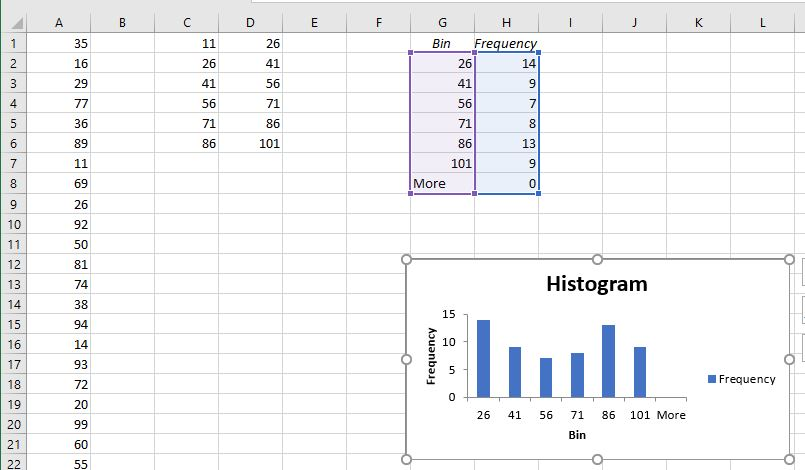The frequencies are in column H.  Now you only need to complete cumulative frequency, relative frequency, and cumulative relative frequency.  In cell I1, enter "Cum Freq."  Then in cell I2, enter =H2.  Next, enter the following formula in cell I3, = I2+H3.  Copy the formula down to cell I8 or enter the following formulas in each of the cells:

• In I4, enter =I3+H4;
• In I5, enter =I4+H5;
• In I6, enter =I5+H6;
• In I7, enter =I6+H7; and
• Finally in I8, enter =I7+H8.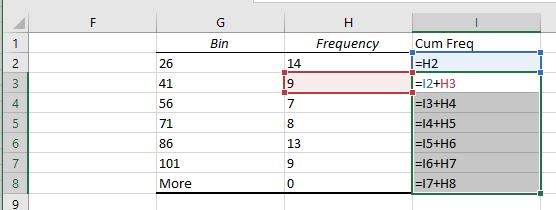The image above shows the formulas in each cell; you will not see this image in the actual Excel spreadsheet what you will see in the image below (14, 23, 30, 38, 51, 60, and 60).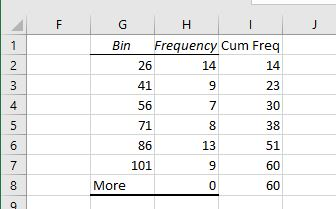The next column to complete is the relative frequencies.  In cell J1, enter Rel Freq.  The total of all the frequencies is 60.  Therefore, enter the formula into cell J2, =H2/60.  Enter the formulas into cells J3 through J8.

• In J3, enter =H3/60;
• In J4, enter =H4/60;
• In J5, enter =H5/60;
• In J6, enter =H6/60;
• In J7, enter =H7/60; and
• Finally in J8, enter =H8/60.The final column to complete is the cumulative relative frequencies.  In cell K1, enter Cum Rel Freq.  The total of all the frequencies is 60.  Therefore, enter the formula into the cell K2, = I2/60.  Enter the formulas into cells K3 through K8.

• In K3, enter =I3/60;
• In K4, enter =I4/60;
• In K5, enter =I5/60;
• In K6, enter =I6/60;
• In K7, enter =I7/60; and
• In K8, enter =I8/60.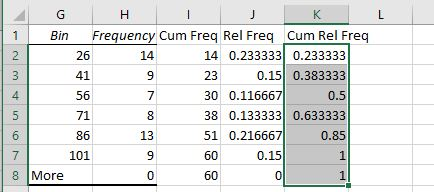The cumulative relative frequencies rounded to six digits is 0.2333, 0.3833, 0.5000, 0.6333, 1.0000,  and 1.0000.

To format the histogram, click on the bars and select Format Data Series.  Change Gap Width to 0%.  Then click the X in the upper right-hand corner.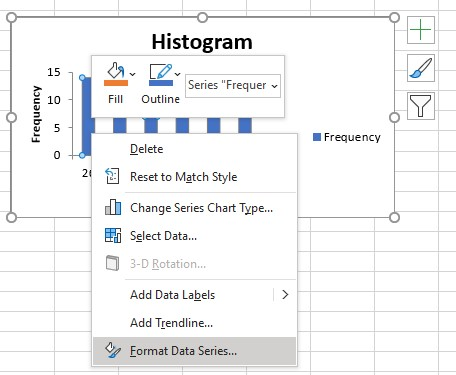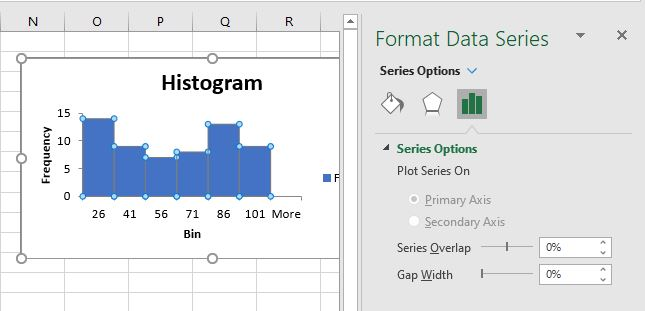### Video on how to create a Frequency Table and Histogram

1.21 Creating a Frequency Table and Histogram in Excel is shared under a not declared license and was authored, remixed, and/or curated by LibreTexts.